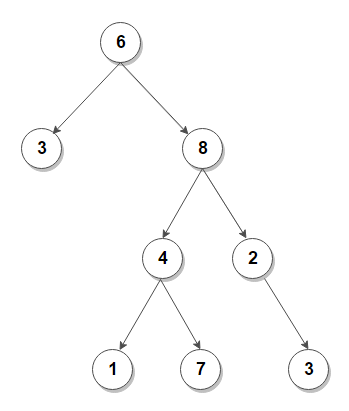# Print corner nodes of every level in binary tree

Given a binary tree, print corner nodes of every level in it.

For example, consider below treeOutput:

6
3 8
4 2
1 3

The idea is very simple. We modify level order traversal of binary tree to maintain level of each node. Then, while doing level order traversal, if the current node happens to be the first node or last node in current level, we simply print it.

C++ implementation –

The time complexity of above solution is O(n) and auxiliary space used by the program is O(n).(1 votes, average: 5.00 out of 5)Loading...